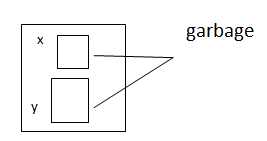Constructors in C++
Ratings:
(4)
Views:643## Constructors

Constructor is a special function in C++ Constructor is used for initialization object auto init of object is done using constructor An object is never created without constructor

### Why Constructor

Data members can’t initialize within the class class A { int x=10; int Y=20; };

• Data members are not initialize within the class
• > object will created with garbage value.

eg : class A { int x; int y; };void main() { A obj1;

#### Data members are public obj1 can be in it at the time creating object

class A { public; int x; int y; } void main() // public data members are not secured

1. class A (    Provide data members cannot initialize at the time of creating object   )

{ int x; int y; }; void main() { A.obj={10.20}; } Statements which has to be executed on creating of object are included inside constructor.

## Types of constructor

Default constructor parameterized constructor Copy constructor

### Properties of constructor in C++(C/S of constructor) - >

Construction name must be the same as class name in C++ Constructor doesn’t have return type not even void It is called automatically at the time of creating object There can be a multiple constructor within the class It can be declared as private, public and protect Constructor are not inherited

### C++ Default constructor

Constructor without any parameters is called default constructor. These are two types of constructors in C++ Compiler defined default constructor User defined default constructor.

### What is difference between Member function and Constructor in C++?

 Member function Constructor Its name should not be the same as class name Its name must be the same as class name It has to be called explicitly It is called implicitly at the time of creating object. It can be called any no.of times on object It is called only once on object. Its operation is modifying and accessing Its operation is initialization They are inherited They are not inherited

### C++ Compiler defined default constructor

If there is no constructor within class, compiler write a constructor without any parameters, This constructor is called default constructor of compiler.### User defined default constructor in C++

It user defined any constructor inside class without any parameters, it is called userdefined default constructor. Example # include<iostream.h> class triangle { float base, height; public : triangle() { base=1.5; height=2.5; } void printf() { cout<<”\n base=”<<base; cout<<”\n  height=”<<height; } };   void main() { triangle triangle1; triangle triangle2; triangle1.print(); triangle2.print(); } o/p : base=1.5 height=2.5 base=1.5 height=2.5 Example # include <iostream.h> class array { int *; public : array(); void read_elements (); void print_elements(); }; array:: array() { a=new int; } void array:: read_elements() { cout<<”\n input elements”; for(int i=0; i<5;i++) cin>>a; }   void array:: print_elements() { cout<<”\n elements are \n”; for(int i=0;i<5;i++) cout<<endl<<a[i]; }   void main() { charname; array subjects; cout<<”\n Enter name”; cin>>name; subjects.read(elements()); cout<<”\n”name; subjects.print_elements();

## Parameterized constructor in C++

Constructor with parameters. It is user defined constructor which receive values of the time of creating object Call send initial values to object by calling the constructor. Example of a parameterized constructor # include<iostream.h> class tiangle { float base, height; public : triangle(float,float); void print() } triangle :: triangle(float b, float h) { base =b; height=h; } void triangle :: print() { cout<< “\n base=”<<base; cout<<”\n height=”<<height } void main() { triangle triangle1(1.5,2.5) triangle triangle2(2.5,3.5) triangle1.print(); triangle2.print(); }

### // wap to create MXN matrix and display

# include<iostream.h> class Matrix { int xxa; int rsize; int csize; public: matrix(int,int); void read_matrix(); void print_matrix(); }; Matrix:: Matrix (int r, int c) { rsize=rc; csize=c; int x[rsize][csize]; a=new int*[rsize]; for(int i=0;i<rsize;i++) *(a+i)=new int[csize]; } void Matrix :: read_matrix() { cout<<”\n input elements of the matrix”; for(int i=0; i<rsize;i++) for(int j=0; j<rsize;j++) cin>>*(*(a+i)+j); cin>>*(*(a+i)+j); } void Matrix :: print_Matrix() { cout<< “\n elements are \n”; for(int i=0;i<rsize;i++) { for(int j=0;j<size;j++) cout<<*(*(a+i)+j)<<”\t”; cout<<endl; } } void main() { Matrix    matrix1(2,2); Matrix    matrix2(3,3); Matrix1.read_matrix(); Matrix1.print_matrix(); Matrix2.read_matrix(); Matrix2.print_matrix(); }     o/p : input elements of the matrix 1       2       3     4 elements are